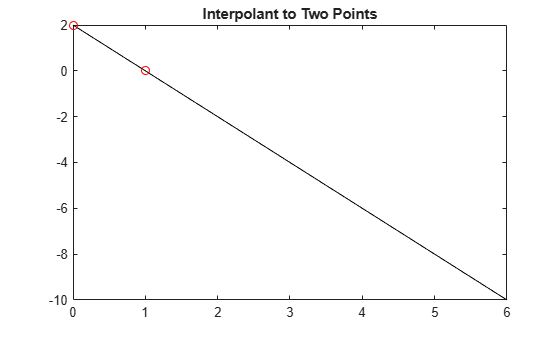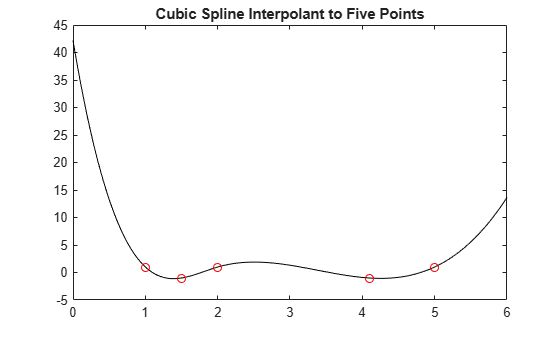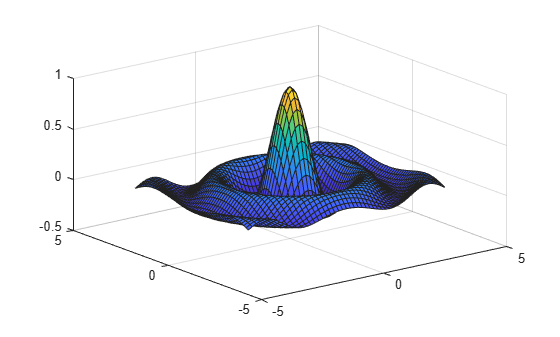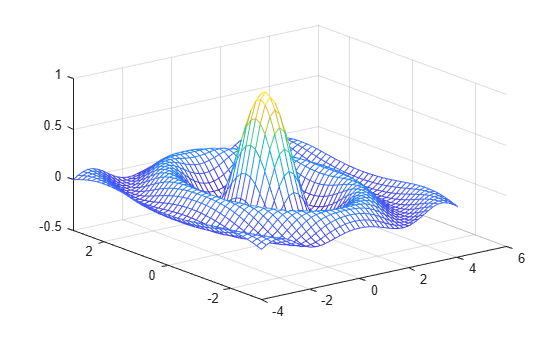# csapi

Cubic spline interpolation

## Syntax

``pp = csapi(x,y)``
``values = csapi(x,y,xx)``

## Description

example

``` NoteFor a simpler but less flexible method to interpolate cubic splines, try the Curve Fitting app or the `fit` function and see About Smoothing Splines. `pp = csapi(x,y)` returns the ppform of a cubic spline s with knot sequence `x` that takes the values `y(:,j)` at `x(j)` for `j=1:length(x)`. The values `y(:,j)` can be scalars, vectors, matrices, and ND-arrays. The function averages the data points with the same data site and then sorts them by their sites. With `x` the resulting sorted data sites, the spline `s` satisfies the not-a-knot end conditions, such as $jum{p}_{x\left(2\right)}{D}_{s}^{3}=0=jum{p}_{x\left(end-1\right)}{D}^{3}\left(s\right)$where D3s is the third derivative of s. If `x` is a cell array of sequences `x1`, ..., `xm` of lengths `n1`, ..., `nm`, then `y` is an array of size `[n1,...,nm]` (or of size `[d,n1,...,nm]` if the interpolant is `d`-valued). In that case, `pp` is the ppform of an `m`-cubic spline interpolant s to such data. In particular, $s\left(x\left({i}_{1}\right),\cdots ,x\left({i}_{m}\right)\right)=y\left(:,{i}_{1},\dots ,{i}_{m}\right)$with ${i}_{1}=1:nl$ and ${i}_{m}=1:nm$.To perform operations on this interpolating cubic spline, such as evaluation, differentiation, plotting, use the pp structure. For more information, see the `fnval`, `fnder`, `fnplt` functions.```

example

````values = csapi(x,y,xx)` returns the values of the smoothing spline evaluated at the points `xx`. This syntax is the same as `fnval(csapi(x,y),xx)`.This command is essentially the MATLAB® function `spline`, which, in turn, is a stripped-down version of the Fortran routine `CUBSPL` in PGS, except that `csapi` (and now also `spline`) accepts vector-valued data and can handle gridded data.```

## Examples

collapse all

This example shows how to use the `csapi` command from Curve Fitting Toolbox™ to construct cubic spline interpolants.

Interpolant to Two Points

The command

`values = csapi(x,y,xx)`

returns the values at `xx` of the cubic spline interpolant to the given data (`x,y`), using the not-a-knot end condition. This interpolant is a piecewise cubic function with break sequence `x`, whose cubic pieces join together to form a function with two continuous derivatives. The "not-a-knot" end condition means that, at the first and last interior break, even the third derivative is continuous (up to round-off error).

Specifying only two data points results in a straight line interpolant.

```x = [0 1]; y = [2 0]; xx = linspace(0,6,121); plot(xx,csapi(x,y,xx),'k-',x,y,'ro') title('Interpolant to Two Points')```Interpolant to Three Points

If you specify three data points, the function outputs a parabola.

```x = [2 3 5]; y = [1 0 4]; plot(xx,csapi(x,y,xx),'k-',x,y,'ro') title('Interpolant to Three Points')```Interpolant to Five Points

More generally, if you specify four or five data points, the function outputs a cubic spline.

```x = [1 1.5 2 4.1 5]; y = [1 -1 1 -1 1]; plot(xx,csapi(x,y,xx),'k-',x,y,'ro') title('Cubic Spline Interpolant to Five Points')```Up to rounding errors, and assuming that `x` is a vector with at least four entries, the statement `pp = csapi``(x,y)` puts the same spline into `pp` as the following statement, except that the description of the spline obtained this second way does not use breaks at `x(2)` and `x(n-1)`:

```pp = fn2fm(spapi(augknt(x([1 3:(end-2) end]),4),x,y),"pp"); ```

As a simple bivariate example, plot a bicubic spline interpolant to a Ricker wavelet.

```x =.0001 + (-4:.2:4); y = -3:.2:3; [yy,xx] = meshgrid(y,x); r = pi*sqrt(xx.^2+yy.^2); z = sin(r)./r; bcs = csapi({x,y},z); fnplt(bcs) axis([-5 5 -5 5 -.5 1])```Since MATLAB® considers the entry `z(i,j)` as the value at (`x(j)`,`y(i)`), the code reverses `x` and `y` in the call to `meshgrid`. The Curve Fitting Toolbox® instead follows the Approximation Theory standard whereas `z(i,j)` is the value at (`x(i)`,`y(j)`).

For this reason, you have to be cautious when you are plotting values of such a bivariate spline with the aid of the MATLAB `mesh` function, as shown here:

```xf = linspace(x(1),x(end),41); yf = linspace(y(1),y(end),41); mesh(xf,yf,fnval(bcs,{xf,yf}).')```Note the use of the transpose of the matrix of values obtained from `fnval`.

## Input Arguments

collapse all

Data sites of data values `y` to be fit, specified as a vector or as a cell array for multivariate data. Spline f is created with knots at each data site `x` such that f(`x(j)`) = `y(:,j)` for all values of `j`.

For multivariate, gridded data, you can specify `x` as a cell array that specifies the data site in each variable dimension: f(`x1(i),x2(j),...xn(k)`) = `y(:,i,j,...,k)`.

Data Types: `single` | `double`

Data values to fit during creation of the spline, specified as a vector, matrix, or array. Data values `y(:,j)` can be scalars, matrices, or n-dimensional arrays. Data values given at the same data site `x` are averaged.

Data Types: `single` | `double`

Evaluation points over which the spline is evaluated, specified as a vector or as a cell array of vectors for multivariate data. Spline evaluation is performed using `fnval`.

Data Types: `single` | `double`

## Output Arguments

collapse all

Spline in ppform, returned as a structure with these fields. For more information on ppform, see The ppform.

Form of the spline, returned as `pp`. `pp` indicates that the spline is given in piecewise polynomial form.

Knot positions of the spline, returned as a vector or as a cell array of vectors for multivariate data. Vectors contain strictly increasing elements that represent the start and end of each of the intervals over which the polynomial pieces are defined.

Coefficients of polynomials for each piece, returned as a matrix or as an array for multivariate data.

Number of polynomial pieces describing the spline, returned as a scalar or as a vector of numbers of pieces in each variable for multivariate data.

Order of the polynomial function describing each polynomial piece of the spline, returned as a scalar or as a vector containing the order in each variable for multivariate data.

Dimensionality of the target function, returned as a scalar.

Evaluated spline, returned as a vector or as a matrix or array for multivariate data. The spline is evaluated at the given evaluation points `xx`.

## Algorithms

`csapi` is an implementation of the Fortran routine `CUBSPL` from PGS.

The algorithm constructs and solves the relevant tridiagonal linear system using the MATLAB sparse matrix capability.

The algorithm also uses the not-a-knot end condition, forcing the first and second polynomial piece of the interpolant to coincide, as well as the second-to-last and the last polynomial piece.